# EMF Equation of DC Generator

Posted on

The derivation of EMF equation for DC generator has two parts:

1. Induced EMF of one conductor
2. Induced EMF of the generator

As the armature rotates, a voltage is generated in its coils. In the case of a generator, the emf of rotation is called the Generated emf or Armature emf and is denoted as Er = Eg.

# EMF Equation of DC Generator

In the case of a motor, the emf of rotation is known as Back emf or Counter emf and represented as Er = Eb.The expression for emf is same for both the operations. I.e., for Generator as well as for Motor.

## Derivation for Induced EMF of One Armature Conductor

For one revolution of the conductor,

Let,

• Φ = Flux produced by each pole in weber (Wb), and
• P = number of poles in the DC generator.
• Z – Total number of armature conductors.
• N – Speed of armature in revolution per minute (r.p.m).
• A – Number of parallel paths in the armature winding.

Therefore,

Total flux produced by all the poles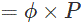And,

Time taken to complete one revolution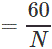Where:

N = speed of the armature conductor in rpm.

Now, according to Faraday’s law of induction, the induced emf of the armature conductor is denoted by “e” which is equal to rate of cutting the flux.

Therefore,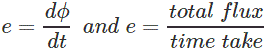Induced emf of one conductor is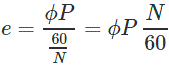## Derivation for Induced EMF for DC Generator

Let us suppose there are Z total numbers of conductor in a generator, and arranged in such a manner that all parallel paths are always in series.

Here,

Z = total numbers of conductor

A = number of parallel paths

Then,

Z/A = number of conductors connected in series

We know that induced emf in each path is same across the line

Therefore,

Induced emf of DC generator

E = emf of one conductor × number of conductor connected in series.

Induced emf of DC generator is: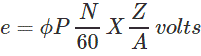Simple wave wound generator

Numbers of parallel paths are only 2 = A

Therefore,

Induced emf for wave type of winding generator is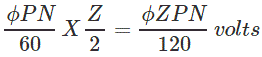Simple lap-wound generator

Here, number of parallel paths is equal to number of conductors in one path

i.e. P = A

Therefore,

Induced emf for lap-wound generator is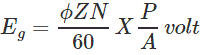### EMF Equation of DC Generator Conclusion

After going through the above portion of emf equation of dc generator we can now establish a derivation for induced EMF of one armature conductor and dc generator. I hope you enjoy when reading this article, thank you.